# lecture 3 Slides.pptx

28. May 2023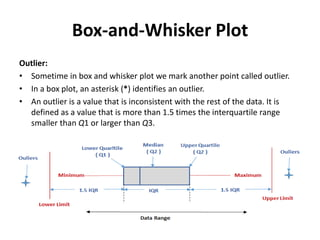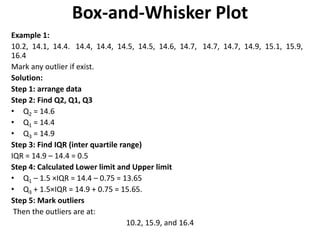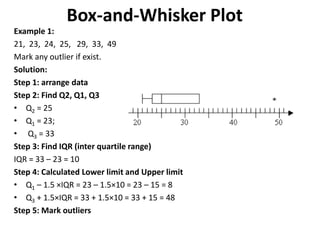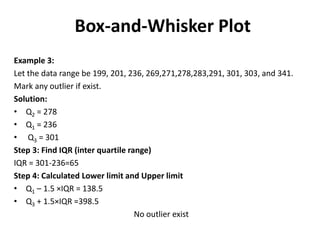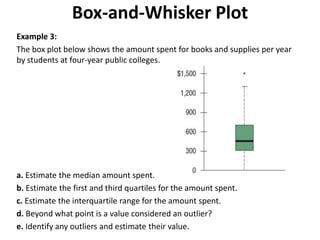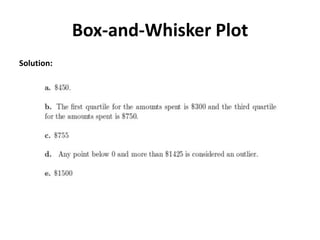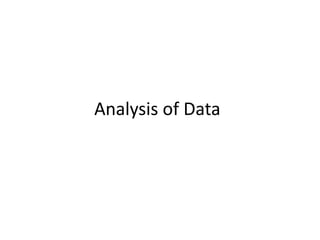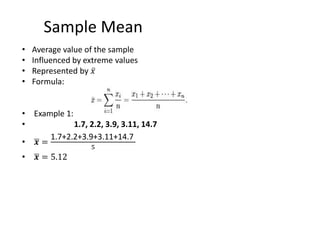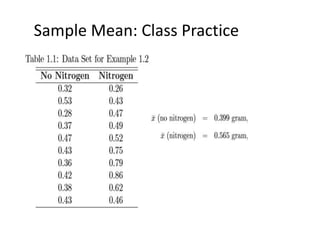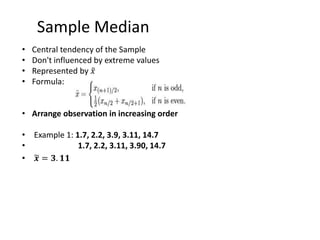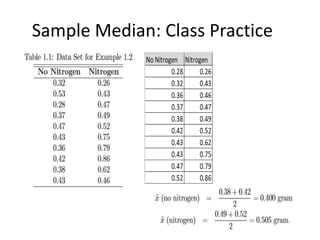1 von 13

### lecture 3 Slides.pptx

• 1. Lecture 3 Topics: Outlier in Box Plot Mean, Median and Spread data SADAF SALEEM Department of Computer Sciences GIFT University, Pakistan Course Title: Probability Theory Course Code: MATH-313 Program: BS MATH Semester: Spring 2023
• 2. Box-and-Whisker Plot Outlier: • Sometime in box and whisker plot we mark another point called outlier. • In a box plot, an asterisk (*) identifies an outlier. • An outlier is a value that is inconsistent with the rest of the data. It is defined as a value that is more than 1.5 times the interquartile range smaller than Q1 or larger than Q3.
• 3. Box-and-Whisker Plot Example 1: 10.2, 14.1, 14.4. 14.4, 14.4, 14.5, 14.5, 14.6, 14.7, 14.7, 14.7, 14.9, 15.1, 15.9, 16.4 Mark any outlier if exist. Solution: Step 1: arrange data Step 2: Find Q2, Q1, Q3 • Q2 = 14.6 • Q1 = 14.4 • Q3 = 14.9 Step 3: Find IQR (inter quartile range) IQR = 14.9 – 14.4 = 0.5 Step 4: Calculated Lower limit and Upper limit • Q1 – 1.5 ×IQR = 14.4 – 0.75 = 13.65 • Q3 + 1.5×IQR = 14.9 + 0.75 = 15.65. Step 5: Mark outliers Then the outliers are at: 10.2, 15.9, and 16.4
• 4. Box-and-Whisker Plot Example 1: 21, 23, 24, 25, 29, 33, 49 Mark any outlier if exist. Solution: Step 1: arrange data Step 2: Find Q2, Q1, Q3 • Q2 = 25 • Q1 = 23; • Q3 = 33 Step 3: Find IQR (inter quartile range) IQR = 33 – 23 = 10 Step 4: Calculated Lower limit and Upper limit • Q1 – 1.5 ×IQR = 23 – 1.5×10 = 23 – 15 = 8 • Q3 + 1.5×IQR = 33 + 1.5×10 = 33 + 15 = 48 Step 5: Mark outliers
• 5. Box-and-Whisker Plot Example 3: Let the data range be 199, 201, 236, 269,271,278,283,291, 301, 303, and 341. Mark any outlier if exist. Solution: • Q2 = 278 • Q1 = 236 • Q3 = 301 Step 3: Find IQR (inter quartile range) IQR = 301-236=65 Step 4: Calculated Lower limit and Upper limit • Q1 – 1.5 ×IQR = 138.5 • Q3 + 1.5×IQR =398.5 No outlier exist
• 6. Box-and-Whisker Plot Example 3: The box plot below shows the amount spent for books and supplies per year by students at four-year public colleges. a. Estimate the median amount spent. b. Estimate the first and third quartiles for the amount spent. c. Estimate the interquartile range for the amount spent. d. Beyond what point is a value considered an outlier? e. Identify any outliers and estimate their value.
• 9. Sample Mean • Average value of the sample • Influenced by extreme values • Represented by 𝑥 • Formula: • Example 1: • 1.7, 2.2, 3.9, 3.11, 14.7 • 𝒙 = 1.7+2.2+3.9+3.11+14.7 5 • 𝒙 = 5.12
• 10. Sample Mean: Class Practice
• 11. Sample Median • Central tendency of the Sample • Don't influenced by extreme values • Represented by 𝑥 • Formula: • Arrange observation in increasing order • Example 1: 1.7, 2.2, 3.9, 3.11, 14.7 • 1.7, 2.2, 3.11, 3.90, 14.7 • 𝒙 = 𝟑. 𝟏𝟏